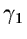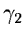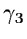Next: Commands Description Up: The wavelet context in Previous: The wavelet context in

## Introduction

A wavelet package concerning two dimensional imaging has been implemented in MIDAS. This package contains several wavelet transform algorithms, specific tools for the wavelet transform, visualisation commands, and three applications of the use of the wavelet transform: filtering, comparison, and deconvolution. These commands can be called if the wavelet context has been initialized by:
set/context wavelet
Table 14.1 shows the available commands.

Table 14.1: Midas commands
 Commands Description TRANSF/WAVE Image Wavelet [Algo] [Nbr_Scale] [Fc] creates the wavelet transform of an image RECONS/WAVE Wavelet Rec_Image reconstructs an image from its wavelet transform HEADER/WAVE Wavelet gives information about a wavelet transform INFO/WAVE Wavelet gives information about each scale of a wavelet transform EXTRAC/WAVE Wavelet Image_Out Scale_Number creates an image from a scale of the wavelet transform ENTER/WAVE Wavelet_in Image_in Scale_Number Wavelet_out replaces a scale of a wavelet transform by an image VISUAL/WAVE Wavelet [Visu_Type] visualizes a wavelet transform with default parameters VISUAL/CUBE Wavelet [output_file] [Disp] [Visu_Mode] [Display] visualizes a wavelet transform in a cube VISUAL/CONT Wavelet [Graphic_Number] [Visu_Mode] [Contour_Level] visualizes the contours of a wavelet transform VISUAL/SYNT Wavelet [output_file] [Display_Number] [Display] creates a visualization image from a wavelet transform VISUAL/PLAN Wavelet [Display] display each scale of the wavelet transform in a window VISUAL/PERS Wavelet [out_file] [Disp_N] [Visu_Mode] [Incr] [Thres] [Display] visualizes in perpsective a wavelet transform FILTER/WAVE I_In I_Out [Algo] [T_Filter] [Iter_Nbr] [N_Scale] [N_Sigma] [Noise] filters an image by using the wavelet transform COMPAR/WAVE Imag_1 Imag_2 [N_Scal] [N_Sigma] [T_Cor] [T_Snr] [Disp] [Init] compares two images in the wavelet space PLOT/SNR Tab_Snr plots the SNR table resulting from the comparison PLOT/COR Tab_Correl plots the correlation resulting from the comparison TUTORIAL/WAVE visualizes the wavelet transform of the galaxy NGC2997 with several algorithms DIRECT/WAVE Imag_In Psf Imag_Out [Nb_Scales] [,,,...] deconvolution with a multiresolution Tichonov's Regularisation CITTERT/WAVE Im_In Psf Im_Out [Resi] [Scal, Iter] [N_Sig, Noise] [Eps] [Fwhm] deconvolution by the regularized Van Cittert's algorithm GRAD/WAVE Im_In Psf Im_Out [Resi] [Nb_Scales] [N_Sigma, Noise] [Eps] [Max_Iter] deconvolution by the regularized one-step gradient algorithm LUCY/WAVE Im_In Psf Im_Out [Resi] [Nb_Scales] [N_Sigma, Noise] [Eps] [Max_Iter] deconvolution by the regularized Lucy's algorithm TRAN1D/WAVE Im_In Wave_Out [Num_Trans] [Num_Line] [Channel] [Nu] one dimensional wavelet transform REC1D/WAVE Wave_in Im_out [Num_Trans] [Channel] [Nu0] reconstructs a 1D signal from its wavelet transformNext: Commands Description Up: The wavelet context in Previous: The wavelet context in
Petra Nass
1999-06-15